Language: Deutsch English

Last Update: 2019 - 05 - 06# Access - Calculated columns in Queries

A key difference between Access and Excel is the use of calculations in Tables (Sheets in Excel). In an Access database you should see a table as a simple storage container for data. If you need to do calculations based on data in the table, you should do that in a query based on that table. You can then display the data of the query, including the calculated fields, in a form or report.

In this article I’m going to show you the basics of creating calculated columns in queries. I’ll show you how to use simple calculations directly in the query as well as calling a custom VBA-Function to do the calculation.

## Simple calculations in Access queries

You can use Expressions in queries. An Expression is like a formula that will produce a result in the context of the running query. An Expression can be a simple mathematical calculation, but likewise a function call of one or even several nested functions.

The simplest possible query with a calculation is this:

SELECT 1 + 1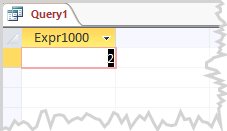This query can be only written and executed in the SQL View of a query. The graphical query designer requires at least one table as data source for the query.

Usually you want to calculate based on the data in a table anyway. So the simple sample above is rather far-fetched.

A very realistic, but still pretty simple calculation, would be the calculation of a gross price based on the net price stored in a table. The relevant column in my demo table is named “NetPrice”. I can use this column name to refer to this column in an Expression.

The formula for to determine the gross price is [NetPrice] + [NetPrice] / 100 * 20. (20 is the current UK VAT rate.) You can use exactly this formula as an expression for a calculated field in an Access query. Just enter it instead of a column name as Field in the Access Query Designer.

That’s going to look like this: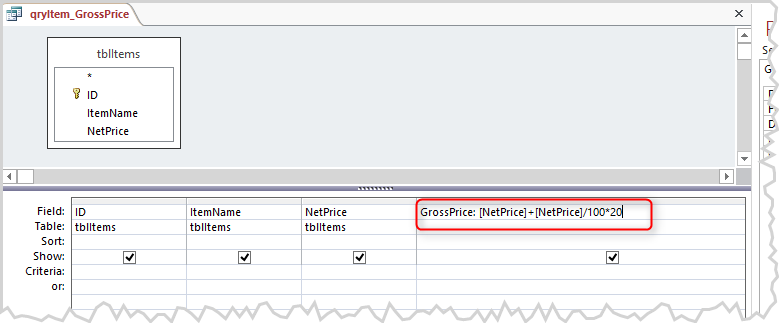The text “GrossPrice” followed by a colon in front of our Expression defines an alias name for this column in the result view of the query. This is just a plain text to identify the column. I could have written “WhatsItsName:” or nothing at all and the result of the calculation would be all the same. If we do not define any name at for the column, Access will do that for us and will name it “Expr1000” or similar. – I strongly recommend you always define an alias name for any calculated column.

In SQL the same query can be written like this:

SELECT ID, ItemName, NetPrice, [NetPrice] + [NetPrice] / 100 * 19 AS GrossPrice FROM tblItems;

The term “AS GrossPrice” is the definition of the alias name for this column in the results.

Here are the results of this query: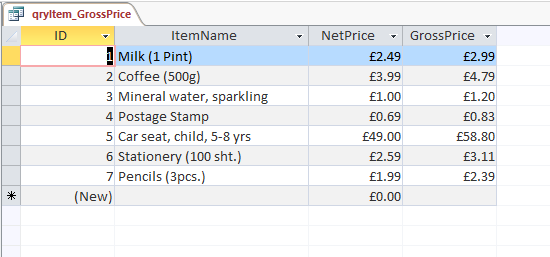So far so good. Technically ok, but…

In the UK for example, there is not just one flat VAT rate on all goods. So our calculation is wrong from a domain knowledge point of view. There are goods with a reduced VAT rate (5%) and some goods (e.g. postage stamps) and services with a 0% VAT rate. – You are still required to charge the 0% VAT though.

We should store the applicable VAT rate in the database table as well. To keep the example simple I add an additional column to the articles table, which stores the VAT rate per article. - In a real database this simple solution would not be ideal.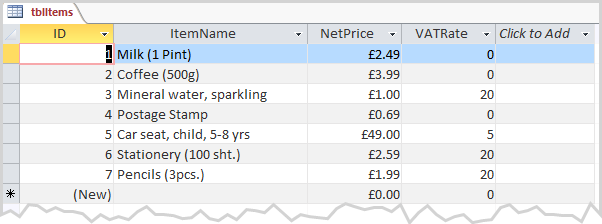The improved expression is looking like this then: [NetPrice] + [NetPrice] / 100 * [VATRate]. We enter this adjusted formula into the query field in the Access Query Designer.

As I used the datatype Number/Decimal for the new column VATRate, Access does not know to format the result of the Expression automatically as currency anymore. To achieve that, I explicitly set the format to currency for this column in the Property Sheet. - In most circumstances I would rather implement such formatting logic in the UI (form or report) than in a query.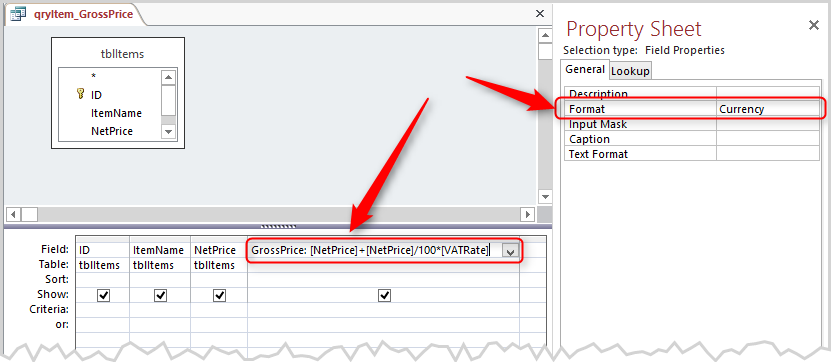And here is the result: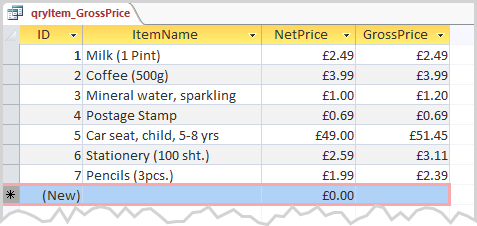The column VATRate does not need to be displayed in the results to be used in the calculation. I left it out of the sample to demonstrate this.

If you use the name of other columns in an Expression, the values to calculate the result will always be those of the current record. If you need the values of another record in your calculation, you’ll have to use function to retrieve the required value. – That’s something I’m going to show in another article someday.

### Video Tutorial on Calculated Fields in Queries

The content of this part of the article, using expressions for calculations, is also available as a video tutorial. You can watch it here.

## Functions in Queries

To roundup this article, I’m going to show you how to use a custom function inside your query. To keep things simple, I write a simple function that is just doing the calculation of the gross price once again.

To do this, I create a new VBA Module and write our function in it.

Two things are important here:

1. The module must not have the same name as the function itself.
2. You can only use Public functions from a normal module (opposed to a Class Module) directly inside a query.

I name my function CalculateGrossPrice. I can call this function inside a query by using this name.

Here is the function: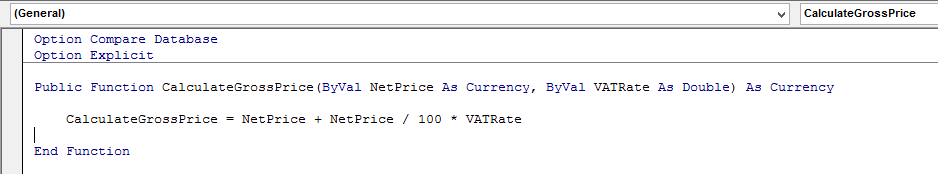In the Query Designer the arguments for the function written inside brackets behind the functions name and are separated by a comma. The complete Expression for the function call is:

CalculateGrossPrice ([NetPrice],[VATRate])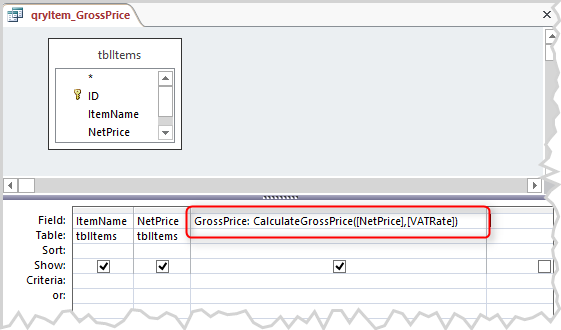The SQL code for this query is this:

SELECT ID, ItemName, NetPrice, CalculateGrossPrice ([NetPrice],[VATRate]) AS GrossPrice FROM tblItems;

The results of this query should look exactly the same as with the previous query containing the complete calculation inside the query.

You would use the exact same approach to call any of the countless built-in functions of Access and VBA inside a query. You are not at all limited to mathematical calculations, but can use any function that has text based arguments and a return value that can be represented as text.

### Video Tutorial on Functions in Queries

The content of this part of the article, using VBA functions for calculations, will be available as a video tutorial soon. You can watch it here as soon as it is published.